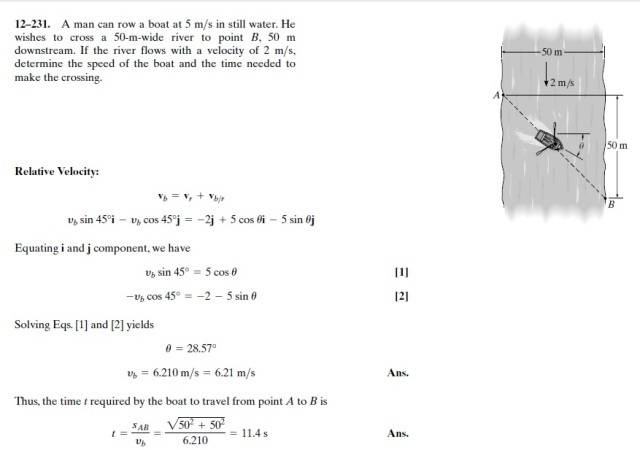# Kinematics Book Question -- Rowing a boat across a river at an angle

raniero
Hi, I was trying to solve the following problem which was assigned to me in my Kinematics course.I found this solution for it. I understand the concept of relative velocity of the boat to the flowing river but what I really cannot understand is the trig part, of Vb/r :
Vb/r = 5cos(theta)i - 5sin(theta)j

Where did the cos(theta) and sin (theta) come from? I understand that this is represented in vectorial form, but I cannot visualize the direction of this velocity. Probably a graphical solution would solve my problem.

## Answers and Replies

Homework Helper
Gold Member
What is the "5?" What are the definitions of "sin" and of "cos?"

raniero
5 is the velocity relative to the 2m/s flow and sin is a function that calculates the fraction out of the hypotenuse value, in this case 5, in a particular direction.

I can now understand the Vb/r part, but I got confused when I tried to visualise Vb .

Any help, especially graphical help, would be greatly appreciated.

Thanks

https://fbcdn-sphotos-h-a.akamaihd.net/hphotos-ak-xpa1/v/t34.0-12/10850742_10205179152815788_535294229_n.jpg?oh=7b9336761d53fdc41bde227b3f48da6e&oe=548A2ED6&__gda__=1418263047_41c23a5855f862274f068da0c46029b4

Homework Helper
vb is at 45 degrees because the boat travels 50 m (width of the river) and lands 50 m downstream from A

Note that the angle between vr and vb is not ##\theta## as I think I read in your drawing....

raniero
According to the solutions it is not θ' as shown in my diagram, but I do not know of another way to represent it graphically. I simply cannot understand what is going on in the equations.

Harshit puri
First of all try to understand directions i denotes in the right direction x axis and j to positive y(upwards) hece all ur movements are in y are downwards hence negative j.
now it makes θ angle with horizontal so cos θ is positive i direction and Sinθ of that vector point downwards it becomes negative j as per their axis so here vb/r represents velocity of boat wrt river (when river is not flowing) to calculate total velocity of body vb u have to add velocity of river vr to it which is -2 (going downwards).
u do 1 thing reverse j direction downwards and solve eqns again it will give u a more clear idea

raniero
I understood this part, what I do not understand is vb = vb sin(45)i - vb cos(45)j

sin(45) = opposite / adjacent = j/i

therefore, adjacent * sin (45) = opposite, but opposite is supposed to be in the j direction opposing the above equation ..
Similarly for cos(45) can be seen.. (should be in the i direction)